# Partition Set Into 2 Subsets With Min Absolute Sum Diff (DP- 16)

Problem Statement:

Partition A Set Into Two Subsets With Minimum Absolute Sum Difference

We are given an array ‘ARR’ with N positive integers. We need to partition the array into two subsets such that the absolute difference of the sum of elements of the subsets is minimum.

We need to return only the minimum absolute difference of the sum of elements of the two partitions.

Examples:

```Example 1: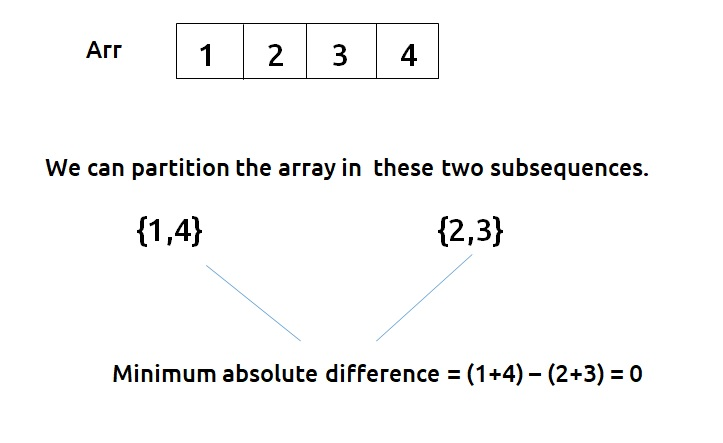```
```Example 2: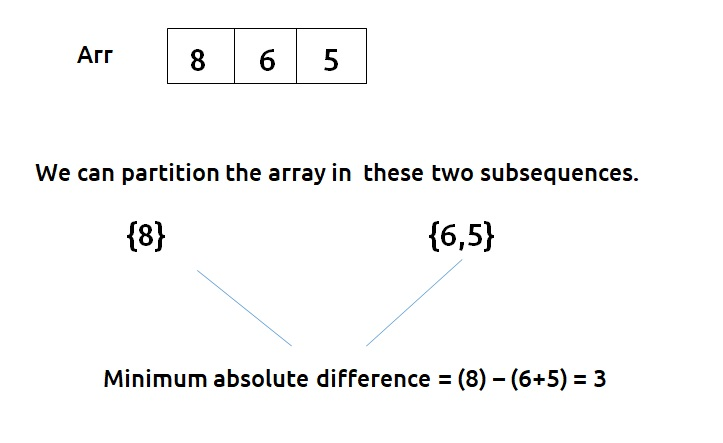```

Disclaimer: Don’t jump directly to the solution, try it out yourself first.

### Solution :

This question is a slight modification of the problem discussed in the Subset Sum equal to target

Before discussing the approach for this question, it is important to understand what we did in the previous question of the Subset Sum equal to the target. There we found whether or not a subset exists in an array with a given target sum. We used a dp array to get to our answer.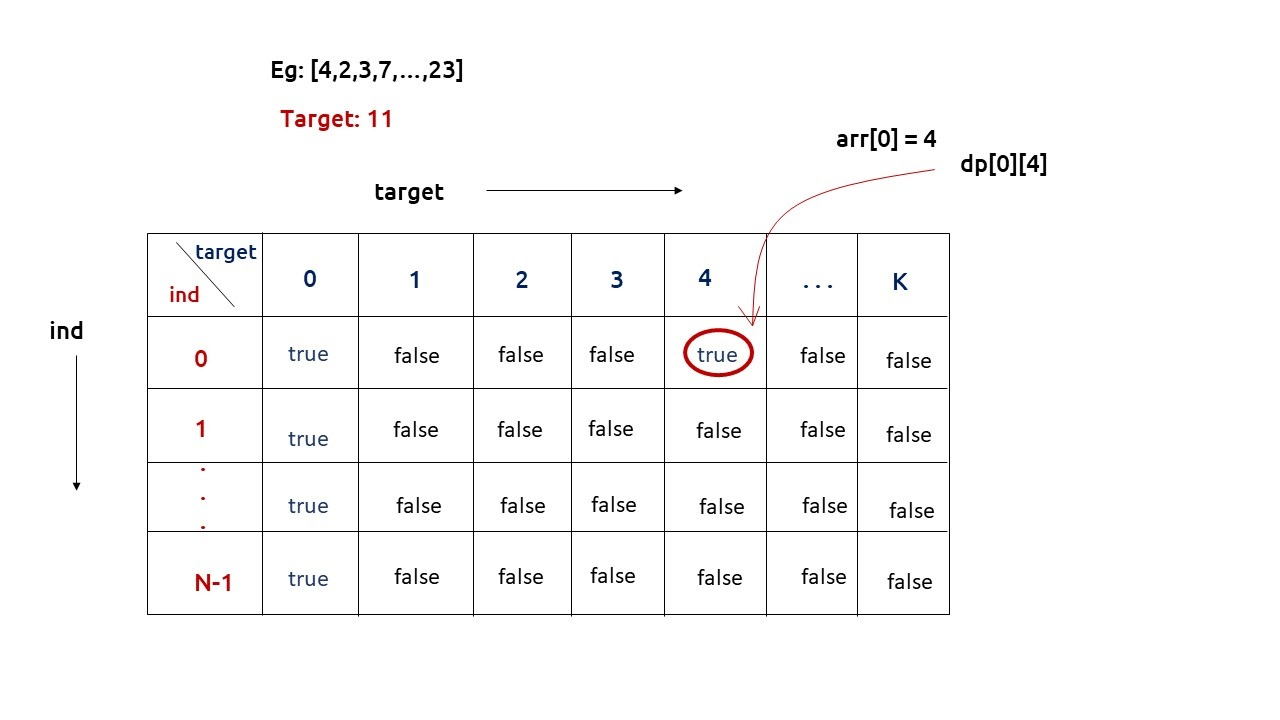We used to return dp[n-1][k] as our answer. One interesting thing that is happening is that for calculating our answer for dp[n-1][k], we are also solving multiple sub-problems and at the same time storing them as well. We will use this property to solve the question of this article.

In this question, we need to partition the array into two subsets( say with sum S1 and S2) and we need to return the minimized absolute difference of S1 and S2. But do we need two variables for it? The answer is No. We can use a variable totSum, which stores the sum of all elements of the input array, and then we can simply say S2 = totSum – S1. Therefore we only need one variable S1.

Now, what values can S1 take? Well, it can go anywhere from 0 (no elements in S1) to totSum( all elements in S1). If we observe the last row of the dp array which we had discussed above, it gives us the targets for which there exists a subset. We will set its column value to totSum, to find the answer from 0(smaller limit of S1) to totSum (the larger limit of S1).

Our work is very simple, using the last row of the dp array, we will first find which all S1 values are valid. Using the valid S1 values, we will find S2 (totSum – S1). From this S1 and S2, we will find their absolute difference. We will return the minimum value of this absolute difference as our answer.

From here we will try to find a subsequence in the array with a target as discussed in Subset Sum equal to target after generating the dp array, we will use the last row to find our answer.

Note: Readers are highly advised to watch this video “Recursion on Subsequences” to understand how we generate subsequences using recursion.

Steps to form the recursive solution:

We will first form the recursive solution by the three points mentioned in Dynamic Programming Introduction

Step 1: Express the problem in terms of indexes.

The array will have an index but there is one more parameter “target”. We are given the initial problem to find whether there exists in the whole array a subsequence whose sum is equal to the target.

So, we can say that initially, we need to find(n-1, target) which means that we need to find whether there exists a subsequence in the array from index 0 to n-1, whose sum is equal to the target. Similarly, we can generalize it for any index ind as follows: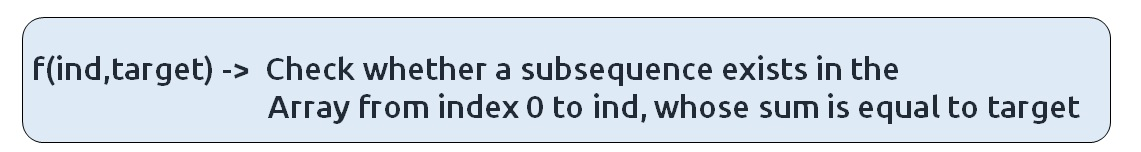Base Cases:

• If target == 0, it means that we have already found the subsequence from the previous steps, so we can return true.
• If ind==0, it means we are at the first element, so we need to return arr[ind]==target. If the element is equal to the target we return true else false.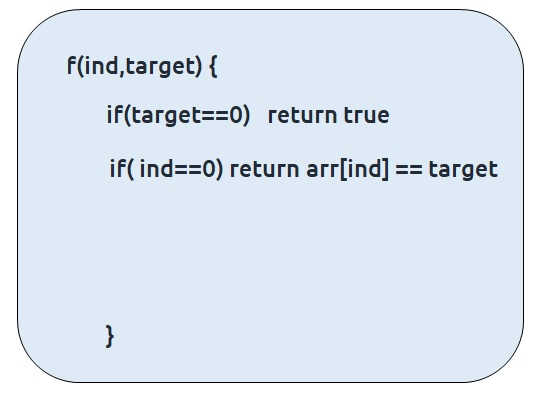Step 2: Try out all possible choices at a given index.

We need to generate all the subsequences. We will use the pick/non-pick technique as discussed in this video “Recursion on Subsequences”.

We have two choices:

• Exclude the current element in the subsequence: We first try to find a subsequence without considering the current index element. For this, we will make a recursive call to f(ind-1,target).
• Include the current element in the subsequence: We will try to find a subsequence by considering the current index as element as part of subsequence. As we have included arr[ind], the updated target which we need to find in the rest if the array will be target – arr[ind]. Therefore, we will call f(ind-1,target-arr[ind]).

Note: We will consider the current element in the subsequence only when the current element is less or equal to the target.

Step 3:  Return (taken || notTaken)

As we are looking for only one subset, if any of the one among taken or not taken returns true, we can return true from our function. Therefore, we return ‘or(||)’ of both of them.

The final pseudocode after steps 1, 2, and 3:

Steps to memoize a recursive solution:

If we draw the recursion tree, we will see that there are overlapping subproblems. In order to convert a recursive solution the following steps will be taken:

1. Create a dp array of size [n][totSum+1]. The size of the input array is ‘n’, so the index will always lie between ‘0’ and ‘n-1’. The target can take any value between ‘0’ and ‘totSum’. Therefore we take the dp array as dp[n][totSum+1]
2. We initialize the dp array to -1.
3. Whenever we want to find the answer of particular parameters (say f(ind,target)), we first check whether the answer is already calculated using the dp array(i.e dp[ind][target]!= -1 ). If yes, simply return the value from the dp array.
4. If not, then we are finding the answer for the given value for the first time, we will use the recursive relation as usual but before returning from the function, we will set dp[ind][target] to the solution we get.
5. When we get the dp array, we will use its last row to find the absolute minimum difference of two partitions.

Code:

## C++ Code

``````#include <bits/stdc++.h>

using namespace std;

bool subsetSumUtil(int ind, int target, vector < int > & arr, vector < vector
< int >> & dp) {
if (target == 0)
return dp[ind][target]=true;

if (ind == 0)
return dp[ind][target] = arr == target;

if (dp[ind][target] != -1)
return dp[ind][target];

bool notTaken = subsetSumUtil(ind - 1, target, arr, dp);

bool taken = false;
if (arr[ind] <= target)
taken = subsetSumUtil(ind - 1, target - arr[ind], arr, dp);

return dp[ind][target] = notTaken || taken;
}

int minSubsetSumDifference(vector < int > & arr, int n) {

int totSum = 0;

for (int i = 0; i < n; i++) {
totSum += arr[i];
}

vector < vector < int >> dp(n, vector < int > (totSum + 1, -1));

for (int i = 0; i <= totSum; i++) {
bool dummy = subsetSumUtil(n - 1, i, arr, dp);
}

int mini = 1e9;
for (int i = 0; i <= totSum; i++) {
if (dp[n - 1][i] == true) {
int diff = abs(i - (totSum - i));
mini = min(mini, diff);
}
}
return mini;

}

int main() {

vector < int > arr = {1,2,3,4};
int n = arr.size();

cout << "The minimum absolute difference is: " << minSubsetSumDifference(arr, n);

}
``````

Output:

The minimum absolute difference is: 0

Time Complexity: O(N*totSum) +O(N) +O(N)

Reason: There are two nested loops that account for O(N*totSum), at starting we are running a for loop to calculate totSum and at last a for loop to traverse the last row.

Space Complexity: O(N*totSum) + O(N)

Reason: We are using an external array of size ‘N * totSum’ and a stack space of O(N).

Steps to convert Recursive Solution to Tabulation one.

To convert the memoization approach to a tabulation one, create a dp array with the same size as done in memoization. We can set its type as bool and initialize it as false.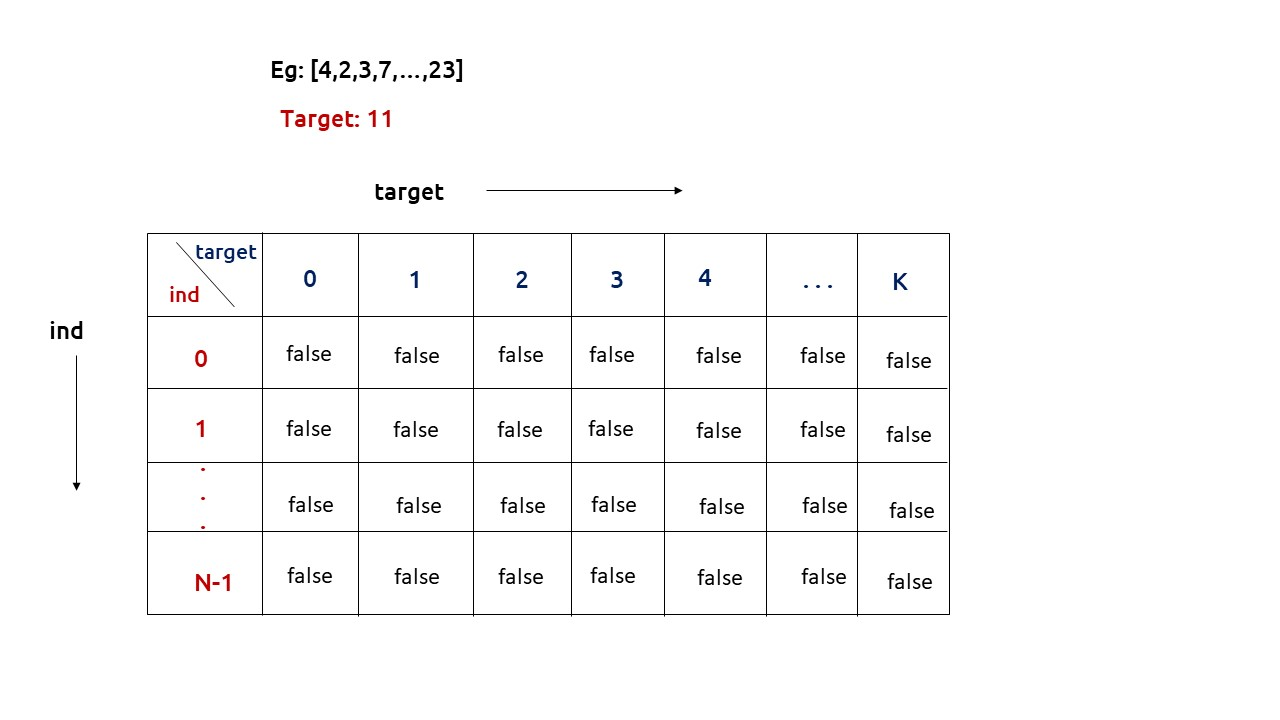First, we need to initialize the base conditions of the recursive solution.

• If target == 0, ind can take any value from 0 to n-1, therefore we need to set the value of the first column as true.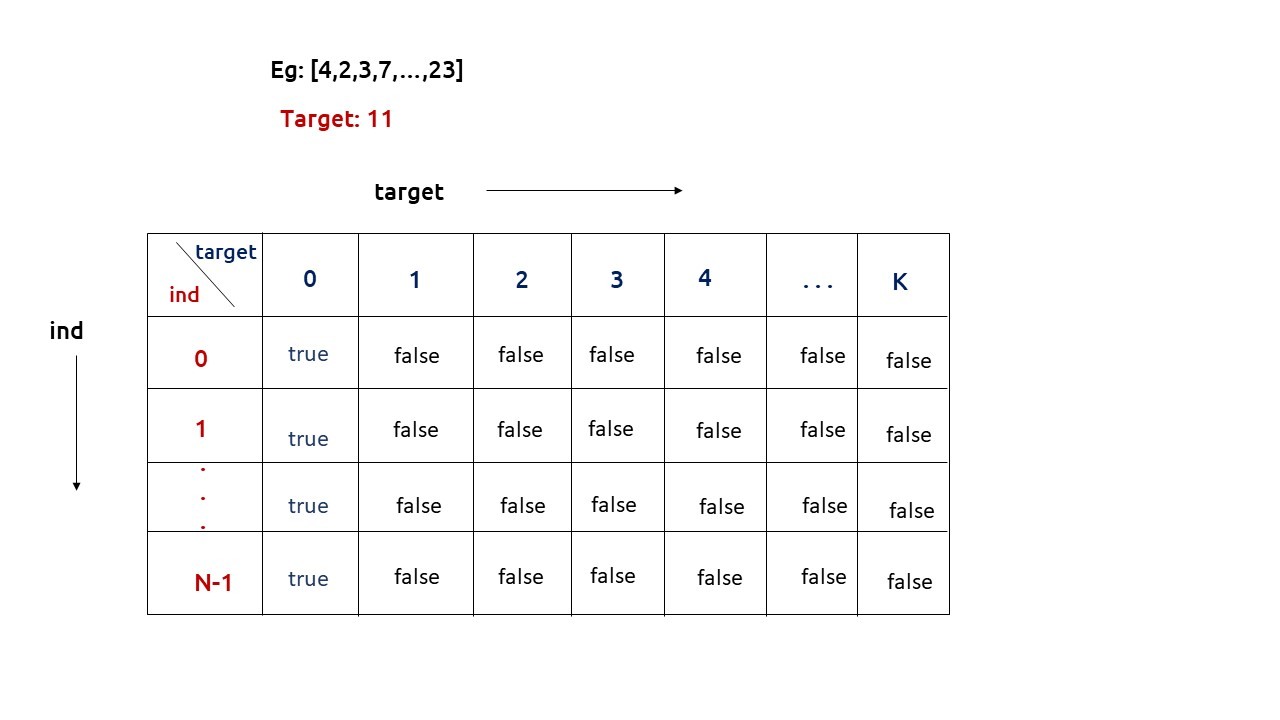• The first row dp[] indicates that only the first element of the array is considered, therefore for the target value equal to arr, only cell with that target will be true, so explicitly set dp[arr] =true, (dp[arr] means that we are considering the first element of the array with the target equal to the first element itself). Please note that it can happen that arr>target, so we first check it: if(arr<=target) then set dp[arr] = true.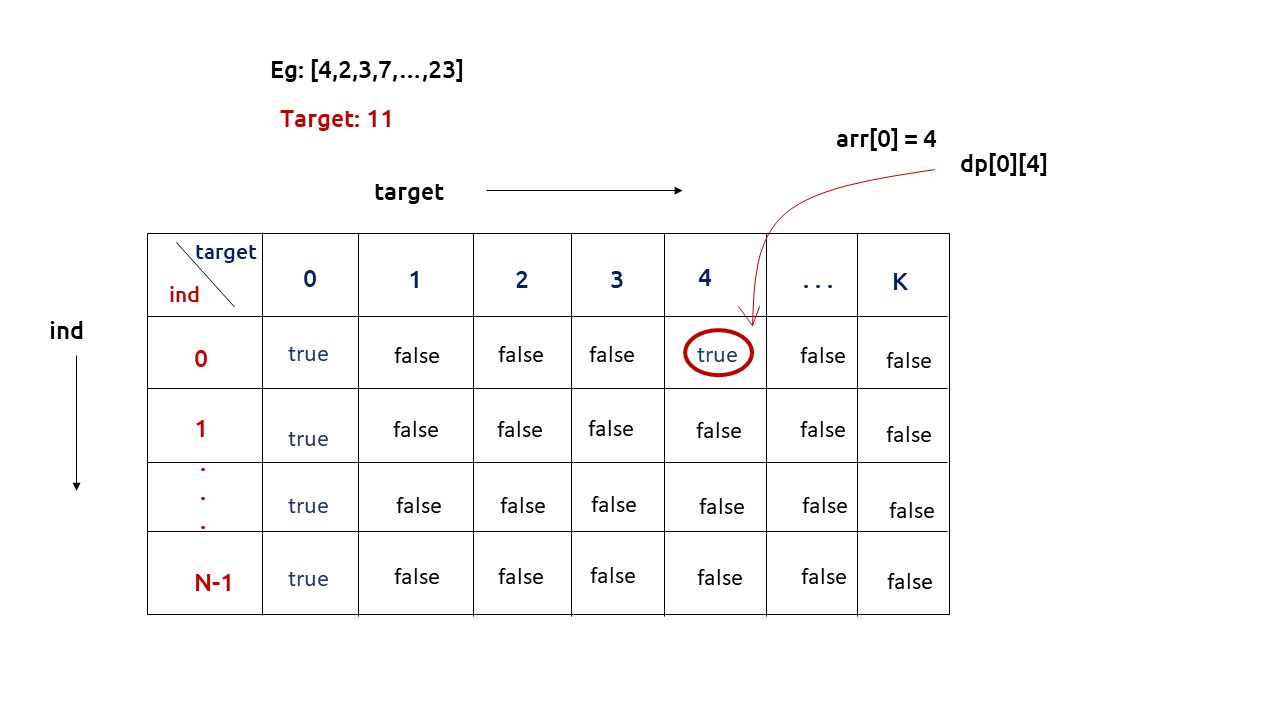• After that , we will set our nested for loops to traverse the dp array and following the logic discussed in the recursive approach, we will set the value of each cell. Instead of recursive calls, we will use the dp array itself.
• When we get the dp array, we will use its last row to find the absolute minimum difference of two partitions.

Code:

## C++ Code

``````#include <bits/stdc++.h>

using namespace std;

int minSubsetSumDifference(vector < int > & arr, int n) {
int totSum = 0;

for (int i = 0; i < n; i++) {
totSum += arr[i];
}

vector < vector < bool >> dp(n, vector < bool > (totSum + 1, false));

for (int i = 0; i < n; i++) {
dp[i] = true;
}

if (arr <= totSum)
dp[totSum] = true;

for (int ind = 1; ind < n; ind++) {
for (int target = 1; target <= totSum; target++) {

bool notTaken = dp[ind - 1][target];

bool taken = false;
if (arr[ind] <= target)
taken = dp[ind - 1][target - arr[ind]];

dp[ind][target] = notTaken || taken;
}
}

int mini = 1e9;
for (int i = 0; i <= totSum; i++) {
if (dp[n - 1][i] == true) {
int diff = abs(i - (totSum - i));
mini = min(mini, diff);
}
}
return mini;
}

int main() {

vector<int> arr = {1,2,3,4};
int n = arr.size();

cout << "The minimum absolute difference is: " << minSubsetSumDifference(arr, n);
}
``````

Output:

The minimum absolute difference is: 0

Time Complexity: O(N*totSum) +O(N) +O(N)

Reason: There are two nested loops that account for O(N*totSum), at starting we are running a for loop to calculate totSum and at last a for loop to traverse the last row.

Space Complexity: O(N*totSum)

Reason: We are using an external array of size ‘N * totSum’. Stack Space is eliminated.

Part 3: Space Optimization

If we closely look the relation,

dp[ind][target] =  dp[ind-1][target] || dp[ind-1][target-arr[ind]]

We see that to calculate a value of a cell of the dp array, we need only the previous row values (say prev). So, we don’t need to store an entire array. Hence we can space optimize it.

Note: Whenever we create a new row ( say cur), we need to explicitly set its first element is true according to our base condition.

Code:

## C++ Code

``````#include <bits/stdc++.h>

using namespace std;

int minSubsetSumDifference(vector < int > & arr, int n) {
int totSum = 0;

for (int i = 0; i < n; i++) {
totSum += arr[i];
}

vector < bool > prev(totSum + 1, false);

prev = true;

if (arr <= totSum)
prev[arr] = true;

for (int ind = 1; ind < n; ind++) {
vector < bool > cur(totSum + 1, false);
cur = true;
for (int target = 1; target <= totSum; target++) {
bool notTaken = prev[target];

bool taken = false;
if (arr[ind] <= target)
taken = prev[target - arr[ind]];

cur[target] = notTaken || taken;
}
prev = cur;
}

int mini = 1e9;
for (int i = 0; i <= totSum; i++) {
if (prev[i] == true) {
int diff = abs(i - (totSum - i));
mini = min(mini, diff);
}
}
return mini;
}

int main() {

vector<int> arr = {1,2,3,4};
int n = arr.size();

cout << "The minimum absolute difference is: " << minSubsetSumDifference(arr, n);
}
``````

Output:

The minimum absolute difference is: 0

Time Complexity: O(N*totSum) +O(N) +O(N)

Reason: There are two nested loops that account for O(N*totSum), at starting we are running a for loop to calculate totSum and at last a for loop to traverse the last row.

Space Complexity: O(totSum)

Reason: We are using an external array of size ‘totSum+1’ to store only one row.

Special thanks to Anshuman Sharma for contributing to this article on takeUforward. If you also wish to share your knowledge with the takeUforward fam, please check out this article